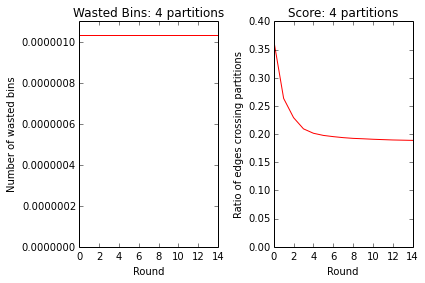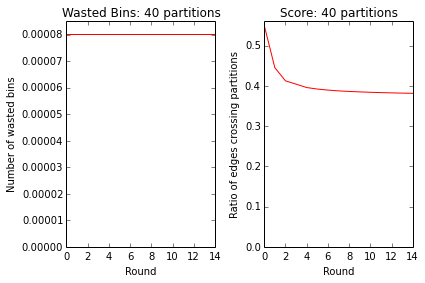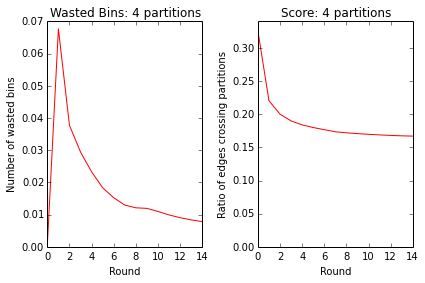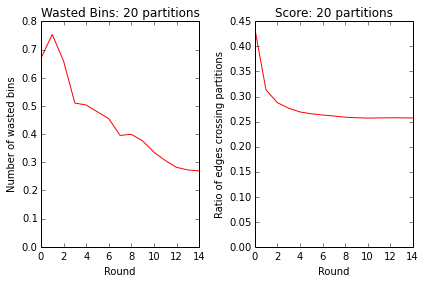In :
# Constants for you to change
import os

DATA_DIRECTORY = '/home/data/justin_files/livejournal'
LIVEJOURNAL_FILENAME = os.path.join(DATA_DIRECTORY, 'livejournal.txt.gz')
LIVEJOURNAL_URL = 'http://snap.stanford.edu/data/soc-LiveJournal1.txt.gz'

# Just have these hardcoded, though we don't really need to
NUM_EDGES = 68993773
NUM_NODES = 4847571
In :

import urllib.request

assert os.path.exists(DATA_DIRECTORY)

# Get your data. This file is ~250M so it will take a bit
if not os.path.exists(LIVEJOURNAL_FILENAME):
urllib.request.urlretrieve(LIVEJOURNAL_URL, LIVEJOURNAL_FILENAME)

# Show a little bit of the file
print('\nFILE STATS:')
!ls -lh $LIVEJOURNAL_FILENAME print('\nSTARTING LINES') !zcat$LIVEJOURNAL_FILENAME | head -n 30

FILE STATS:
-rw-rw-r-- 1 justinvf justinvf 248M Dec 13 14:19 /home/data/justin_files/livejournal/livejournal.txt.gz

STARTING LINES

gzip: stdout: Broken pipe
In :
# Cleaning the data

import numpy as np
import gzip

def row_generator():
"""This will generate all the edges in the graph."""
with gzip.open(LIVEJOURNAL_FILENAME, 'rt') as f:
for line in f:
if line.startswith('#'):
continue
else:
(left_node, right_node) = line.split('\t')
yield(int(left_node), int(right_node))

def to_undirected(edge_iterable, num_edges, num_nodes, shuffle=True):
"""Takes an iterable of edges and produces the list of edges for the undirected graph.

> to_undirected([[0,1],[1,2],[2,10]], 3, 11)
array([[ 0,  1],
[ 1,  0],
[ 1,  2],
[ 2,  1],
[ 2, 10],
[10,  2]])
"""
# need int64 to do gross bithacks
as_array = np.zeros((num_edges, 2), dtype=np.int64)
for (i, (n_0, n_1)) in enumerate(edge_iterable):
as_array[i,0] = n_0
as_array[i,1] = n_1
# The graph is directed, but we want to make it undirected,
# which means we will duplicate some rows.

left_nodes = as_array[:,0]
right_nodes = as_array[:,1]

if shuffle:
the_shuffle = np.arange(num_nodes)
np.random.shuffle(the_shuffle)
left_nodes = the_shuffle.take(left_nodes)
right_nodes = the_shuffle.take(right_nodes)

# numpy.unique will not unique whole rows, so this little bit-hacking
# is a quick way to get unique rows after making a flipped copy of
# each edge.
max_bits = int(np.ceil(np.log2(num_nodes + 1)))

encoded_edges_forward = np.left_shift(left_nodes, max_bits) | right_nodes

# Flip the columns and do it again:
encoded_edges_reverse = np.left_shift(right_nodes, max_bits) | left_nodes

unique_encoded_edges = np.unique(np.hstack((encoded_edges_forward, encoded_edges_reverse)))

left_node_decoded = np.right_shift(unique_encoded_edges, max_bits)

# Mask out the high order bits
right_node_decoded = (2 ** (max_bits) - 1) & unique_encoded_edges

undirected_edges = np.vstack((left_node_decoded, right_node_decoded)).T.astype(np.int32)

# ascontiguousarray so that it's c-contiguous for cython code below
return np.ascontiguousarray(undirected_edges)

def get_clean_data(shuffle=True):
if shuffle:
name = os.path.join(DATA_DIRECTORY, 'LJ-cleaned-shuffled.npy')
else:
name = os.path.join(DATA_DIRECTORY, 'LJ-cleaned.npy')

if os.path.exists(name):
else:
print('Parsing from zip. Will write to file {}'.format(name), flush=True)
# Lets get the edges into one big array
edges = to_undirected(row_generator(), NUM_EDGES, NUM_NODES, shuffle=shuffle)
print('ORIGINAL DIST: {} MIN: {} MAX: {}'.format(np.abs(edges[:,0] - edges[:,1]).mean(), edges.min(), edges.max()))
np.save(name, edges)
return edges
In :
%time edges = get_clean_data(shuffle=True)
%time unshuffled_edges = get_clean_data(shuffle=False)
print('\nEDGES SHAPE: {}'.format(edges.shape))
CPU times: user 0 ns, sys: 212 ms, total: 212 ms
Wall time: 212 ms
CPU times: user 0 ns, sys: 188 ms, total: 188 ms
Wall time: 191 ms

EDGES SHAPE: (86220856, 2)
In :
def score(assignment, edges):
"""Compute the score given an assignment of vertices.

N nodes are assigned to clusters 0 to K-1.

assignment: Vector where N[i] is the cluster node i is assigned to.
edges: The edges in the graph, assumed to have one in each direction

Returns: (total wasted bin space, ratio of edges cut)
"""
balance = np.bincount(assignment) / len(assignment)
waste = (np.max(balance) - balance).sum()

left_edge_assignment = assignment.take(edges[:,0])
right_edge_assignment = assignment.take(edges[:,1])
mismatch = (left_edge_assignment != right_edge_assignment).sum()
cut_ratio = mismatch / len(edges)
return (waste, cut_ratio)
In :
%pylab inline
Populating the interactive namespace from numpy and matplotlib
/home/justinvf/anaconda/envs/blogging/lib/python3.3/site-packages/matplotlib/mathtext.py:46: UserWarning: Due to a bug in pyparsing <= 2.0.0 on Python 3.x, packrat parsing has been disabled.  Mathtext rendering will be much slower as a result.  Install pyparsing 2.0.0 or later to improve performance.
warn("Due to a bug in pyparsing <= 2.0.0 on Python 3.x, packrat parsing "
In :
%%cython
import numpy as np
cimport cython

cdef int UNMAPPED = -1

def linear_deterministic_greedy(int[:,::] edges,
int num_nodes,
int num_partitions,
int[::] partition):
"""
This algorithm favors a cluster if it has many neighbors of a node, but
penalizes the cluster if it is close to capacity.

edges: An [:,2] array of edges.
num_nodes: The number of nodes in the graph.
num_partitions: How many partitions we are breaking the graph into.
partition: The partition from a previous run. Used for restreaming.

Returns: A new partition.
"""
# The output partition

if partition is None:
partition = np.repeat(np.int32(UNMAPPED), num_nodes)

cdef int[::] partition_sizes = np.zeros(num_partitions, dtype=np.int32)

cdef int[::] partition_votes = np.zeros(num_partitions, dtype=np.int32)

# Fine to be a little off, to stay integers
cdef int partition_capacity = num_nodes / num_partitions

cdef int last_left = edges[0,0]
cdef int i = 0
cdef int left = 0
cdef int right = 0
cdef int arg = 0
cdef int max_arg = 0
cdef int max_val = 0
cdef int val = 0
cdef int len_edges = len(edges)

for i in range(len_edges):
left = edges[i,0]
right = edges[i,1]

if last_left != left:
# We have found a new node so assign last_left to a partition

max_arg = 0
partition_capacity - partition_sizes)

for arg in range(1, num_partitions):
partition_capacity - partition_sizes[arg])
if val > max_val:
max_arg = arg
max_val = val

if max_val == 0:
max_arg = arg
# No neighbors (or multiple maxed out) so "randomly" select
# the smallest partition
for arg in range(i % num_partitions, num_partitions):
if partition_sizes[arg] < partition_capacity:
max_arg = arg
max_val = 1
break
if max_val == 0:
for arg in range(0, i % num_partitions):
if partition_sizes[arg] < partition_capacity:
max_arg = arg
break

partition_sizes[max_arg] += 1
partition[last_left] = max_arg
last_left = left

if partition[right] != UNMAPPED:

# Clean up the last assignment
max_arg = 0
max_val = 0
for arg in range(0, num_partitions):
if partition_sizes[arg] < partition_capacity:
1 - partition_sizes[arg] / partition_capacity)
if val > max_val:
max_arg = arg
max_val = val
partition[left] = max_arg

return np.asarray(partition)
In :
def draw_figures(waste_values, edge_score_values, num_partitions):
fig, axes = plt.subplots(nrows=1, ncols=2)

(waste_axes, score_axes) = axes
waste_axes.plot(waste_values, 'r')
waste_axes.set_xlabel('Round')
waste_axes.set_ylabel('Number of wasted bins')
waste_axes.set_ylim(bottom=0)
waste_axes.set_title('Wasted Bins: {} partitions'.format(num_partitions))

score_axes.plot(edge_score_values, 'r')
score_axes.set_xlabel('Round')
score_axes.set_ylabel('Ratio of edges crossing partitions')
score_axes.set_ylim(bottom=0)
score_axes.set_title('Score: {} partitions'.format(num_partitions))

fig.tight_layout()
In :
def run_restreaming_greedy(edges, num_nodes, num_partitions=10, num_iterations=10):
print('\n{} PARTITIONS'.format(num_partitions))
assignments = None
print('ROUND\tWASTE\tSCORE')
waste_values = []
edge_score_values = []
flipped_edges = numpy.flipud(edges).copy()
for i in range(num_iterations):
assignments = linear_deterministic_greedy(edges, NUM_NODES, num_partitions, assignments)
(waste, edge_score) = score(assignments, edges)
waste_values.append(waste)
edge_score_values.append(edge_score)
print('{}\t{:0.3f}\t{:0.3f}'.format(i, waste, edge_score))
draw_figures(waste_values, edge_score_values, num_partitions)
In :
# Use the greedy method to break into 4 partitions
%time run_restreaming_greedy(edges, NUM_NODES, num_partitions=4, num_iterations=15)
4 PARTITIONS
ROUND	WASTE	SCORE
0	0.000	0.367
1	0.000	0.263
2	0.000	0.229
3	0.000	0.209
4	0.000	0.201
5	0.000	0.198
6	0.000	0.195
7	0.000	0.194
8	0.000	0.192
9	0.000	0.192
10	0.000	0.191
11	0.000	0.190
12	0.000	0.190
13	0.000	0.189
14	0.000	0.189
CPU times: user 1min 15s, sys: 6.25 s, total: 1min 21s
Wall time: 1min 21sIn :
# Use the greedy method to break into 20 partitions
%time run_restreaming_greedy(edges, NUM_NODES, num_partitions=40, num_iterations=15)
40 PARTITIONS
ROUND	WASTE	SCORE
0	0.000	0.550
1	0.000	0.445
2	0.000	0.412
3	0.000	0.404
4	0.000	0.396
5	0.000	0.392
6	0.000	0.389
7	0.000	0.387
8	0.000	0.386
9	0.000	0.385
10	0.000	0.384
11	0.000	0.383
12	0.000	0.382
13	0.000	0.382
14	0.000	0.381
CPU times: user 1min 22s, sys: 6.41 s, total: 1min 28s
Wall time: 1min 29sIn :
%%cython
import numpy as np

cdef int UNMAPPED = -1

def fennel(int[:,::] edges,
int num_nodes,
int num_partitions,
int[::] partition,
float alpha):
"""
This algorithm favors a cluster if it has many neighbors of a node, but
penalizes the cluster if it is close to capacity.

partition: A previous partition of the nodes. Set to -1's if a node has not been assigned.
"""

cdef int[::] partition_sizes = None
# The output partition
if partition is None:
partition = np.repeat(np.int32(UNMAPPED), num_nodes)
partition_sizes = np.zeros(num_partitions, dtype=np.int32)
else:
partition_sizes = np.bincount(partition).astype(np.int32)

cdef int[::] partition_votes = np.zeros(num_partitions, dtype=np.int32)

cdef float partition_capacity = num_nodes / num_partitions

cdef int last_left = edges[0,0]
cdef int i = 0
cdef int left = 0
cdef int right = 0
cdef int arg = 0
cdef int max_arg = 0
cdef float max_val = 0
cdef float val = 0
cdef int len_edges = len(edges)
cdef int previous_assignment = 0

for i in range(len_edges):
left = edges[i,0]
right = edges[i,1]

if last_left != left:
# New left node, so we have to assign last left

# Remember placement of last_left in the previous assignment
previous_assignment = partition[last_left]

max_arg = 0
max_val = partition_votes - alpha * partition_sizes
if previous_assignment == 0:
# We remove the node from its current partition before
# deciding to re-add it, so subtract alpha to give
# result of 1 lower partition size.
max_val += alpha

for arg in range(1, num_partitions):
val = partition_votes[arg] - alpha * partition_sizes[arg]
if previous_assignment == arg:
# See comment above
val += alpha
if val > max_val:
max_arg = arg
max_val = val

if max_arg != previous_assignment:
partition[last_left] = max_arg
partition_sizes[max_arg] += 1
if previous_assignment != UNMAPPED:
partition_sizes[previous_assignment] -= 1

last_left = left

if partition[right] != -1:

# TODO: finish off this fencepost....
partition[left] = 0

return np.asarray(partition)
In :
def run_fennel_restreaming(edges, num_nodes, num_partitions, num_iterations):
print('{} PARTITIONS'.format(num_partitions))
assignments = None
# We will explore this parameter in a future post. This is sub-optimal
alphas = np.linspace(1.251608191745264e-07, 7.588951557309824e-05, num_iterations)
waste_scores, edge_score_values = [], []
print('ROUND\tALPHA\tWASTE\tSCORE')
for i in range(num_iterations):
alpha = alphas[i]
assignments = fennel(edges, NUM_NODES, num_partitions, assignments, alpha)
(waste, edge_score) = score(assignments, edges)
print('{}\t{:.1e}\t{:0.3f}\t{:0.3f}'.format(i, alpha, waste, edge_score))
waste_scores.append(waste)
edge_score_values.append(edge_score)
draw_figures(waste_scores, edge_score_values, num_partitions)
In :
%time run_fennel_restreaming(edges, NUM_NODES, 4, 15)
4 PARTITIONS
ROUND	ALPHA	WASTE	SCORE
0	1.3e-07	0.000	0.326
1	5.5e-06	0.068	0.221
2	1.1e-05	0.038	0.200
3	1.6e-05	0.029	0.190
4	2.2e-05	0.023	0.184
5	2.7e-05	0.018	0.180
6	3.3e-05	0.015	0.177
7	3.8e-05	0.013	0.173
8	4.3e-05	0.012	0.172
9	4.9e-05	0.012	0.171
10	5.4e-05	0.011	0.170
11	6.0e-05	0.010	0.169
12	6.5e-05	0.009	0.168
13	7.0e-05	0.008	0.167
14	7.6e-05	0.008	0.167
CPU times: user 1min 14s, sys: 6.47 s, total: 1min 20s
Wall time: 1min 20sIn :
%time run_fennel_restreaming(edges, NUM_NODES, 20, 15)
20 PARTITIONS
ROUND	ALPHA	WASTE	SCORE
0	1.3e-07	0.669	0.437
1	5.5e-06	0.754	0.313
2	1.1e-05	0.660	0.288
3	1.6e-05	0.510	0.277
4	2.2e-05	0.503	0.269
5	2.7e-05	0.479	0.266
6	3.3e-05	0.455	0.263
7	3.8e-05	0.395	0.261
8	4.3e-05	0.399	0.259
9	4.9e-05	0.376	0.258
10	5.4e-05	0.336	0.257
11	6.0e-05	0.306	0.257
12	6.5e-05	0.282	0.258
13	7.0e-05	0.273	0.257
14	7.6e-05	0.270	0.257
CPU times: user 1min 18s, sys: 6.6 s, total: 1min 25s
Wall time: 1min 25sIn [ ]: